# Calculation Nodes and Networks

The .NET Calculation Engine library finaquant® calcs (available until August 2013) will comprise Calculation Nodes and Networks in addition to the table functions of the non-commercial finaquant® protos.

The term table function should be clear by now: Functions with in-memory data tables as input and output parameters.

These table functions can be compared to an extensive library of general-purpose Stored Procedures of a database like Oracle or MySQL, or to FOX formulas of some SAP business applications.

But what are these Calculation Nodes and Networks?

### Calculation Node

A Calculation Node is a single unit (Worker Ant) of a Calculation Network (i.e. network of nodes) that carries a single table function. In compliance with its table function, a node can have multiple input and output tables.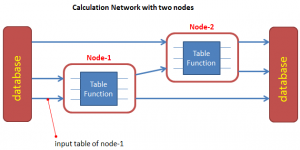Briefly, a Calculation Node can accomplish the following tasks:

1. Reads all its input tables from various data stores, or from the output of a another node.
2. Checks if all input tables are valid.
3. Executes the assigned table function to generate output tables.
4. Checks if all output tables are valid.
5. Writes output tables to the configured data stores. The most typical data store is the generic database as explained in article: https://software.tuncalik.com/persistent-table-array/3330

Note that all input and output tables are associated with an instance parameter, namely Calculation Instance, to differentiate between multiple instances of data tables.

For example, you may want to calculate product prices or dealer commissions for each country and year. A vector with these two attributes (country-year pair) is in this case your Calculation Instance.

Apart from the input tables, a Calculation Instance is the single parameter of a Node or Network.

### Possible Input Sources for a Calculation Node

There are four possible input sources: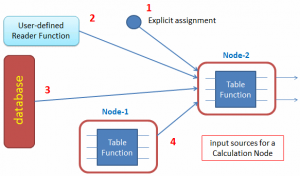1. Explicit (manual) assignment of an input table
2. A user-defined delegate (reader) function
3. Generic database of the calculation engine
4. Output table of another calculation node, usually in a network with multiple nodes

Explicit assignment (1) can be useful for tests and simulations.

A user-defined delegate function (2) can fetch the input table from any data store, increasing the flexibility and complexity of the node.

A relational database (3) is the most typical input source for operational calculations like dealer commissions, performance fees, financial plans and so on.

Output of a calculation node (4) can feed another node in a calculation network.

### Calculation Node Events

User-defined delegate functions can be registered to Calculation Node events like:

• AfterValidatingInputsBeforeNodeExecution (i.e. just before executing the table function)
• AfterNodeExecutionBeforeValidatingOutputs
• AfterValidatingOutputsBeforeWriting (i.e. before writing resultant tables into a data store)
• AfterWritingAllOutputs (i.e. just before the whole node execution is completed)

An example log table of a Calculation Network with multiple nodes: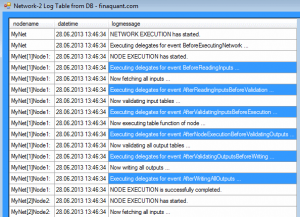These node events can be used for purposes like:

• Writing, manipulating or validating some input tables before the Network or Node is executed
• Triggering workflow functions like sending emails, reports etc.

### Node execution is skipped if all inputs are old

The table function of a Calculation Node is not executed at all if no input table was updated after the last execution of the node.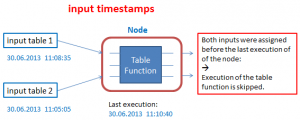This makes sense because outputs will be same if the inputs remain constant unless the configuration (i.e. behavior) of the Calculation Node was changed in the meanwhile. Naturally, all timestamps are reset if the behavior of a node is changed through configuration.

This time-saving feature brings performance advantages in simulations and operational calculations with big data. In a Calculation Network with multiple Nodes, only the Nodes with fresh inputs must be executed to obtain the results.

### Simulations with Calculation Nodes & Networks

Because table-valued functions use in-memory data tables as input and output parameters, a Calculation Network can operate totally detached from a database.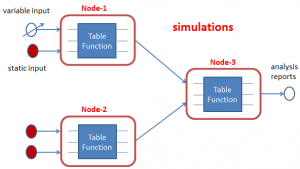Thus, the .net library finaquant® calcs can be used for both operational and analytical calculations.

Once you have configured a Calculation Network for an operational calculation, you can use exactly the same Network for simulations.

The real-time simulation ability makes all sorts of analytical evaluations possible like scenario analysis and optimizations in order to produce strategic information for decision support.

Tunç Ali Kütükçüoglu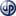Copyright secured by Digiprove © 2013 Tunc Ali Kütükcüoglu
This entry was posted in Calculation engine and tagged , , , . Bookmark the permalink.